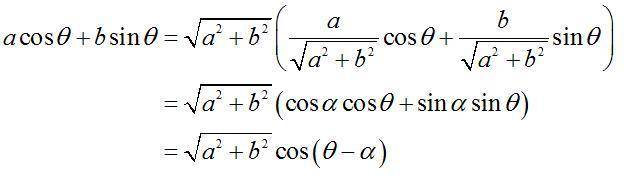# A cos theta + b sin theta

Hey,
I’ve got a test in one week’s time and was studying through my text book of geometry and trigonometry. I came across a “rule” which shows how to simplify expressions in the form of $$a \cos \theta + b \sin \theta$$ but I do not understand how this “rule” works.
The simplify rule:

$$a \cos \theta + b \sin \theta = \sqrt{a^2+b^2} (\frac{a}{\sqrt{ a^2+b^2}} \cos \theta + \frac{b}{ \sqrt{ a^2+b^2}} \sin \theta )$$

$$a \cos \theta + b \sin \theta = \sqrt{a^2+b^2}(\cos \alpha \cos \theta + \sin \alpha \sin \theta)$$

$$a \cos \theta + b \sin \theta = \sqrt{a^2+b^2} \cos (\theta - \alpha)$$

where $$\alpha$$ is an acute angle

In the text book this is explained using a right angle triangle where $$\alpha$$ is the unknown angle being measured, side a is the adjacent side and side b is the opposite, therefore the hypotenuse is equal to $$sqrt{a^2+b^2}$$. However I do not understand this method which the book uses to explain, and was wondering if somebody out there knew how to explain/prove how/where this “rule” has come from. Thank you for any legitimate reply,
Pavadrin

EDIT: those latex code isn't wokring properly so ive attached this image link:Last edited by a moderator:

## Answers and Replies

okay thanks for the link J77